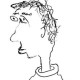# The Newton stratification is true

Let$G$ be a connected reductive group over$\mathbf{Q}_p$, and let$\mu$ be a$G$-valued (geometric) conjugacy class of minuscule cocharacters, with reflex field$E$. In their Annals paper, Caraiani and Scholze defined a very interesting stratification of the flag variety$\mathcal{F}\ell_{G,\mu}$ (regarded as an adic space over$E$) into strata$\mathcal{F}\ell_{G,\mu}^{b}$, where$b$ runs over the Kottwitz set$B(G,\mu^{-1})$. Let me roughly recall how this goes: any (geometric) point$x \to \mathcal{F}\ell_{G,\mu}$ determines a canonical modification$\mathcal{E}_x \to \mathcal{E}_{triv}$ of the trivial$G$-bundle on the Fargues-Fontaine curve, meromorphic at$\infty$ and with “mermorphy$\mu$” in the usual sense. On the other hand, Fargues proved that$G$-bundles on the curve are classified up to isomorphism by$B(G)$, and then Caraiani-Scholze and Rapoport proved that$\mu$-meromorphic modifications of the trivial bundle are exactly classified by the subset$B(G,\mu^{-1})$ (CS proved that only these elements can occur; R proved that all of these elements occur). The Newton stratification just records which element of this set parametrizes the bundle$\mathcal{E}_x$.

The individual strata are pretty weird. For example, if$G=GL_n$ and$\mu=(1,0,\dots,0)$, then$\mathcal{F}\ell_{G,\mu} \simeq \mathbf{P}^{n-1}$ and the open stratum is just the usual Drinfeld space$\Omega^{n-1}$, but the other strata are of the form$\Omega^{n-i-1} \times^{P_{n-i,i}(\mathbf{Q}_p)} GL_n(\mathbf{Q}_p)$, where$P_{n-i,i}$ is the evident parabolic in$GL_n$ and the action on$\Omega^{n-i-1}$ is via the natural map$P_{n-i,i}(\mathbf{Q}_p) \twoheadrightarrow GL_{n-i}(\mathbf{Q}_p)$. Qualitatively, this says that they’re unions of profinitely many copies of lower-dimensional Drinfeld spaces. In particular, the non-open strata are not rigid analytic spaces. There are also examples of strata which don’t have any classical rigid analytic points. However, the$\mathcal{F}\ell_{G,\mu}^{b}$‘s are always perfectly well-defined from the topological or diamond point of view.

Anyway, I’m getting to the following thing, which settles a question left open by Caraiani-Scholze.

Theorem. Topologically, the Newton stratification of$\mathcal{F}\ell_{G,\mu}$ is a true stratification: the closure of any stratum is a union of strata.

The idea is as follows. After base-changing from$E$ to the completed maximal unramified extension$E'$ (which is a harmless move), there is a canonical map$\zeta: \mathcal{F}\ell_{G,\mu,E'} \to \mathrm{Bun}_{G}$ sending$x$ to the isomorphism class of$\mathcal{E}_x$. Here$\mathrm{Bun}_{G}$ denotes the stack of$G$-bundles on the Fargues-Fontaine curve, regarded as a stack on the category of perfectoid spaces over$\overline{\mathbf{F}_p}$. This stack is stratified by locally closed substacks$\mathrm{Bun}_{G}^{b}$ defined in the obvious way, and by construction the Newton stratification is just the pullback of this stratification along$\zeta$. Now, by Fargues’s theorem we get an identification$|\mathrm{Bun}_{G}| = B(G)$, so it is completely trivial to see that the stratification of$\mathrm{Bun}_{G}$ is a true stratification (at the level of topological spaces). We then conclude by the following observation:

Proposition. The map$\zeta$ is universally open.

The idea is to observe that$\zeta$ factors as a composition of two maps$\mathcal{F}\ell_{G,\mu,E'} \to [\mathcal{F}\ell_{G,\mu,E'}/\underline{G(\mathbf{Q}_p)}] \to \mathrm{Bun}_{G}$. Here the first map is a$\underline{G(\mathbf{Q}_p)}$-torsor by construction, so it’s universally open by e.g. Lemma 10.13 here. More subtly, the second map is also universally open. Why? Because it is cohomologically smooth in the sense of Definition 23.8 here; universal openness then follows by Proposition 23.11 in the same document.

For the cohomological smoothness claim, take any affinoid perfectoid space with a map$T \to \mathrm{Bun}_{G}$, corresponding to some bundle$\mathcal{F} / \mathcal{X}_T$. After some thought, one works out the fiber product$X = T \times_{\mathrm{Bun}_{G}} [\mathcal{F}\ell_{G,\mu,E'}/\underline{G(\mathbf{Q}_p)}]$ “explicitly”: it parametrizes untilts of$T$ over$E'$ together with isomorphism classes of$\mu^{-1}$-meromorphic modifications$\mathcal{E}\to \mathcal{F}$ supported along the section$T^{\sharp} \to \mathcal{X}_T$ induced by our preferred untilt, with the property that$\mathcal{E}$ is trivial at every geometric point of$T$. Without the final condition, we get a larger functor$X'$ which etale-locally on$T$ is isomorphic to$T \times_{\mathrm{Spd}(\overline{\mathbf{F}_p})} \mathcal{F}\ell_{G,\mu^{-1},E'}^{\lozenge}$. (To get the latter description, note that etale-locally on$T$ we can trivialize$\mathcal{F}$ on the formal completion of the curve along$T^{\sharp}$, and then use Beauville-Laszlo to interpret the remaining data as a suitably restricted modification of the trivial$G$-torsor on$\mathrm{Spec} \mathbf{B}_{dR}^{+}(\mathcal{O}(T^{\sharp}))$. This is a Schubert cell in a Grassmannian. Then use Caraiani-Scholze’s results on the Bialynicki-Birula map.) Anyway anyway, after a little more fiddling around the point is basically that the projection$X' \to T$ is cohomologically smooth because it’s the base change of a smooth map of rigid spaces. By Kedlaya-Liu plus epsilon, the natural map$X \to X'$ is an open immersion, so$X \to T$ is cohomologically smooth. Since$T$ was arbitrary, this is enough.

## 2 thoughts on “The Newton stratification is true”

1.Your Honors Math B students says:

Cool post, but when are you going to post our practice final?

Like

1.arithmetica says:

Well played.

Like